# Applications of differential equation

14 May 2020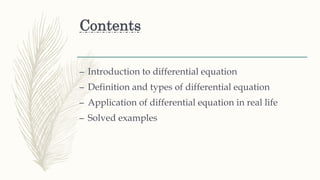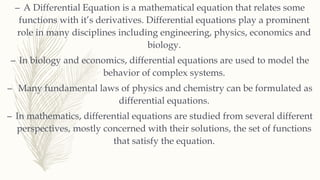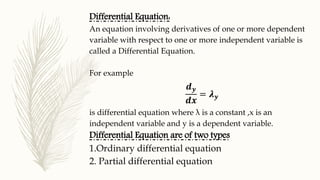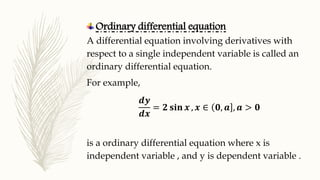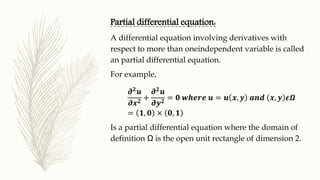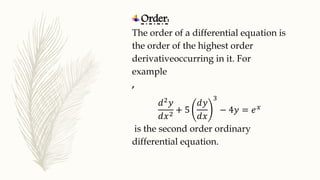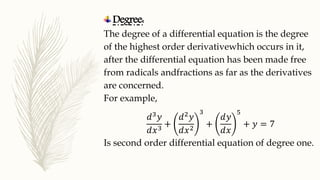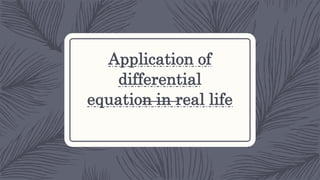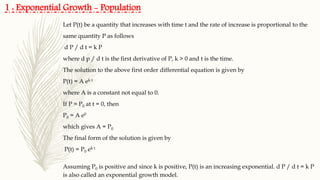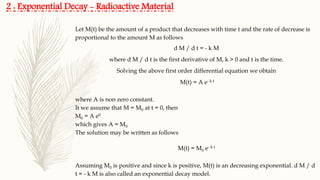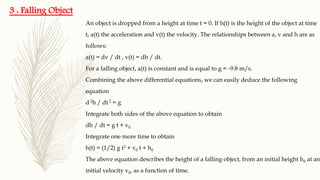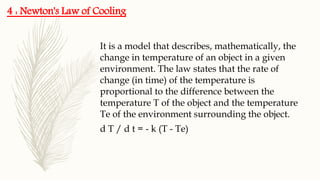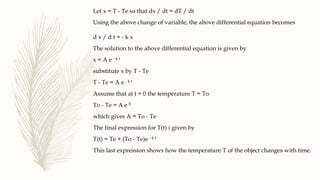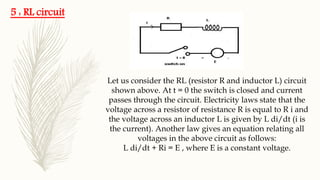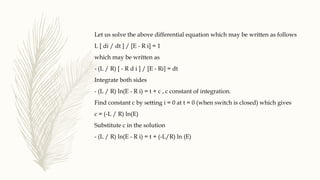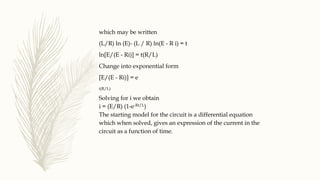1 sur 18

### Applications of differential equation

• 1. Applications of Differential equation
• 2. Contents – Introduction to differential equation – Definition and types of differential equation – Application of differential equation in real life – Solved examples
• 3. Introduction
• 4. – A Differential Equation is a mathematical equation that relates some functions with it’s derivatives. Differential equations play a prominent role in many disciplines including engineering, physics, economics and biology. – In biology and economics, differential equations are used to model the behavior of complex systems. – Many fundamental laws of physics and chemistry can be formulated as differential equations. – In mathematics, differential equations are studied from several different perspectives, mostly concerned with their solutions, the set of functions that satisfy the equation.
• 5. Differential Equation: An equation involving derivatives of one or more dependent variable with respect to one or more independent variable is called a Differential Equation. For example ⅆ 𝒚 ⅆ𝒙 = 𝝀 𝒚 is differential equation where λ is a constant ,x is an independent variable and y is a dependent variable. Differential Equation are of two types 1.Ordinary differential equation 2. Partial differential equation
• 6. Ordinary differential equation A differential equation involving derivatives with respect to a single independent variable is called an ordinary differential equation. For example, ⅆ𝒚 ⅆ𝒙 = 𝟐 𝐬𝐢𝐧 𝒙 , 𝒙 ∈ 𝟎, 𝒂 , 𝒂 > 𝟎 is a ordinary differential equation where x is independent variable , and y is dependent variable .
• 7. Partial differential equation: A differential equation involving derivatives with respect to more than oneindependent variable is called an partial differential equation. For example, 𝝏 𝟐 𝒖 𝝏𝒙 𝟐 + 𝝏 𝟐 𝒖 𝝏𝒚 𝟐 = 𝟎 𝒘𝒉𝒆𝒓𝒆 𝒖 = 𝒖 𝒙, 𝒚 𝒂𝒏ⅆ 𝒙, 𝒚 𝝐𝜴 = 𝟏, 𝟎 × 𝟎, 𝟏 Is a partial differential equation where the domain of definition Ω is the open unit rectangle of dimension 2.
• 8. Order: The order of a differential equation is the order of the highest order derivativeoccurring in it. For example , ⅆ2 𝑦 ⅆ𝑥2 + 5 ⅆ𝑦 ⅆ𝑥 3 − 4𝑦 = ⅇ 𝑥 is the second order ordinary differential equation.
• 9. Degree: The degree of a differential equation is the degree of the highest order derivativewhich occurs in it, after the differential equation has been made free from radicals andfractions as far as the derivatives are concerned. For example, ⅆ3 𝑦 ⅆ𝑥3 + ⅆ2 𝑦 ⅆ𝑥2 3 + ⅆ𝑦 ⅆ𝑥 5 + 𝑦 = 7 Is second order differential equation of degree one.
• 10. Application of differential equation in real life
• 11. Let P(t) be a quantity that increases with time t and the rate of increase is proportional to the same quantity P as follows d P / d t = k P where d p / d t is the first derivative of P, k > 0 and t is the time. The solution to the above first order differential equation is given by P(t) = A ek t where A is a constant not equal to 0. If P = P0 at t = 0, then P0 = A e0 which gives A = P0 The final form of the solution is given by P(t) = P0 ek t Assuming P0 is positive and since k is positive, P(t) is an increasing exponential. d P / d t = k P is also called an exponential growth model. 1 : Exponential Growth - Population
• 12. Let M(t) be the amount of a product that decreases with time t and the rate of decrease is proportional to the amount M as follows d M / d t = - k M where d M / d t is the first derivative of M, k > 0 and t is the time. Solving the above first order differential equation we obtain M(t) = A e- k t where A is non zero constant. It we assume that M = M0 at t = 0, then M0 = A e0 which gives A = M0 The solution may be written as follows M(t) = M0 e- k t Assuming M0 is positive and since k is positive, M(t) is an decreasing exponential. d M / d t = - k M is also called an exponential decay model. 2 : Exponential Decay - Radioactive Material
• 13. An object is dropped from a height at time t = 0. If h(t) is the height of the object at time t, a(t) the acceleration and v(t) the velocity. The relationships between a, v and h are as follows: a(t) = dv / dt , v(t) = dh / dt. For a falling object, a(t) is constant and is equal to g = -9.8 m/s. Combining the above differential equations, we can easily deduce the following equation d 2h / dt 2 = g Integrate both sides of the above equation to obtain dh / dt = g t + v0 Integrate one more time to obtain h(t) = (1/2) g t2 + v0 t + h0 The above equation describes the height of a falling object, from an initial height h0 at an initial velocity v0, as a function of time. 3 : Falling Object
• 14. It is a model that describes, mathematically, the change in temperature of an object in a given environment. The law states that the rate of change (in time) of the temperature is proportional to the difference between the temperature T of the object and the temperature Te of the environment surrounding the object. d T / d t = - k (T - Te) 4 : Newton's Law of Cooling
• 15. Let x = T - Te so that dx / dt = dT / dt Using the above change of variable, the above differential equation becomes d x / d t = - k x The solution to the above differential equation is given by x = A e - k t substitute x by T - Te T - Te = A e - k t Assume that at t = 0 the temperature T = To To - Te = A e 0 which gives A = To - Te The final expression for T(t) i given by T(t) = Te + (To - Te)e - k t This last expression shows how the temperature T of the object changes with time.
• 16. 5 : RL circuit Let us consider the RL (resistor R and inductor L) circuit shown above. At t = 0 the switch is closed and current passes through the circuit. Electricity laws state that the voltage across a resistor of resistance R is equal to R i and the voltage across an inductor L is given by L di/dt (i is the current). Another law gives an equation relating all voltages in the above circuit as follows: L di/dt + Ri = E , where E is a constant voltage.
• 17. Let us solve the above differential equation which may be written as follows L [ di / dt ] / [E - R i] = 1 which may be written as - (L / R) [ - R d i ] / [E - Ri] = dt Integrate both sides - (L / R) ln(E - R i) = t + c , c constant of integration. Find constant c by setting i = 0 at t = 0 (when switch is closed) which gives c = (-L / R) ln(E) Substitute c in the solution - (L / R) ln(E - R i) = t + (-L/R) ln (E)
• 18. which may be written (L/R) ln (E)- (L / R) ln(E - R i) = t ln[E/(E - Ri)] = t(R/L) Change into exponential form [E/(E - Ri)] = e t(R/L) Solving for i we obtain i = (E/R) (1-e-Rt/L) The starting model for the circuit is a differential equation which when solved, gives an expression of the current in the circuit as a function of time.